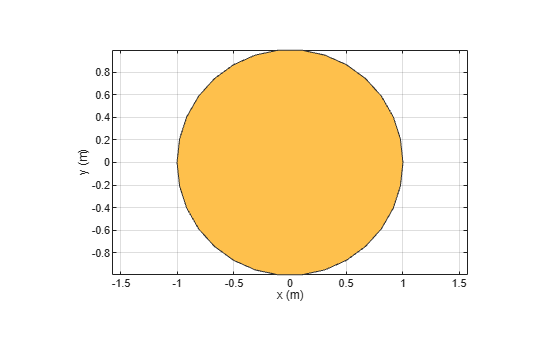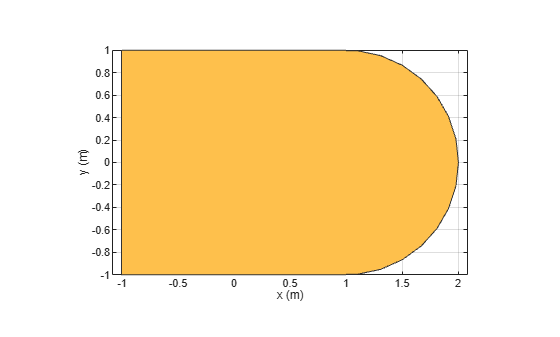# antenna.Circle

Create circle centered at origin on X-Y plane

## Description

Use the `antenna.Circle` object to create a circle centered at the origin and on the X-Y plane. You can use `antenna.Polygon` to create single-layer or multi-layered antennas using `pcbStack`.

## Creation

### Syntax

``circle = antenna.Circle``
``circle = antenna.Circle(Name=Value)``

### Description

example

````circle = antenna.Circle` creates a circle centered at the origin and on the X-Y plane.```

example

````circle = antenna.Circle(Name=Value)` sets properties using one or more name-value arguments. `Name` is the property name and `Value` is the corresponding value. You can specify several name-value arguments in any order as `Name1`= `Value1`, `...`, `NameN`=`ValueN`. Properties not specified retain their default values.```

## Properties

expand all

Name of circle, specified as a string or a character vector.

Example: `"Circle1"`

Data Types: `char` | `string`

Cartesian coordinates of center of circle, specified as a 2-element vector with each element measured in meters.

Example: `[0.006 0.006]`

Data Types: `double`

Circle radius, specified as a scalar in meters.

Example: `2`

Data Types: `double`

Number of discretization points on circumference, specified as a scalar.

Example: `16`

Data Types: `double`

## Object Functions

 `add` Boolean unite operation on two shapes `subtract` Boolean subtraction operation on two shapes `intersect` Boolean intersection operation on two shapes `plus` Shape1 + Shape2 `minus` Shape1 - Shape2 `and` Shape1 & Shape2 `area` Calculate area of shape in square meters `show` Display antenna, array structures or shapes `plot` Plot boundary of shape `mesh` Mesh properties of metal, dielectric antenna, or array structure `rotate` Rotate shape about axis and angle `rotateX` Rotate shape about x-axis and angle `rotateY` Rotate shape about y-axis and angle `rotateZ` Rotate shape about z-axis and angle `translate` Move shape to new location `scale` Change the size of the shape by a fixed amount `mirrorX` Mirror shape along X-axis `mirrorY` Mirror shape along Y-axis `removeHoles` Remove holes from shape `removeSlivers` Remove sliver outliers from boundary of shape

## Examples

collapse all

Create and view circle using `antenna.Circle` and view it.

`c1 = antenna.Circle`
```c1 = Circle with properties: Name: 'mycircle' Center: [0 0] Radius: 1 NumPoints: 30 ```
`show(c1)`Create a circle centered at the origin with a radius of 4 m.

`c2 = antenna.Circle(Radius=4)`
```c2 = Circle with properties: Name: 'mycircle' Center: [0 0] Radius: 4 NumPoints: 30 ```

Create a circle with a radius of 1m. The center of the circle is at [1 0].

`circle1 = antenna.Circle(Center=[1 0],Radius=1);`

Create a rectangle with a length of 2m and a width of 4m centered at the origin.

`rect1 = antenna.Rectangle(Length=2,Width=2);`

Add the two shapes together using the `+` function.

`polygon1 = circle1+rect1`
```polygon1 = Polygon with properties: Name: 'mypolygon' Vertices: [21x3 double] ```
`show(polygon1)`## Version History

Introduced in R2017a